Probability

# Data Presentation - Histogram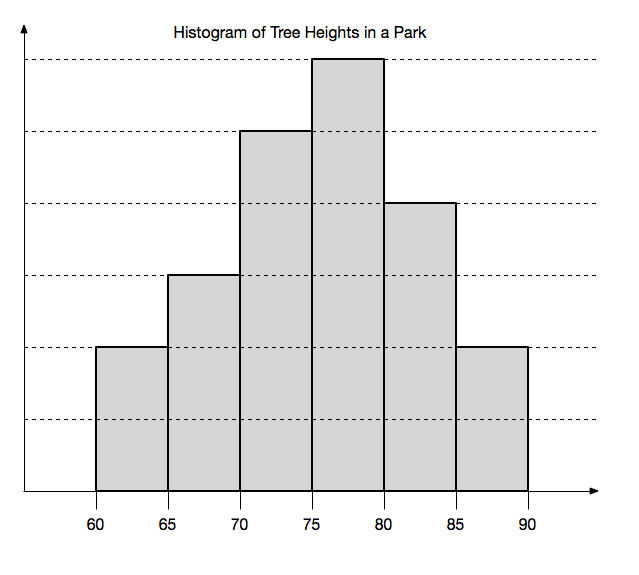The above histogram shows the height of trees (in feet) in a park. If there are 12 trees that have a height of $85$ to $90$ feet, how many trees are there in the park?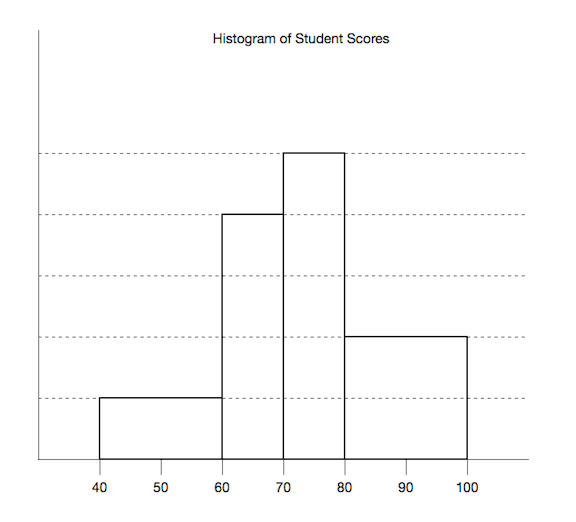The above histogram shows the test scores of students in a class. If 28 students scored from 40 to 60, how many students scored between 70 to 80?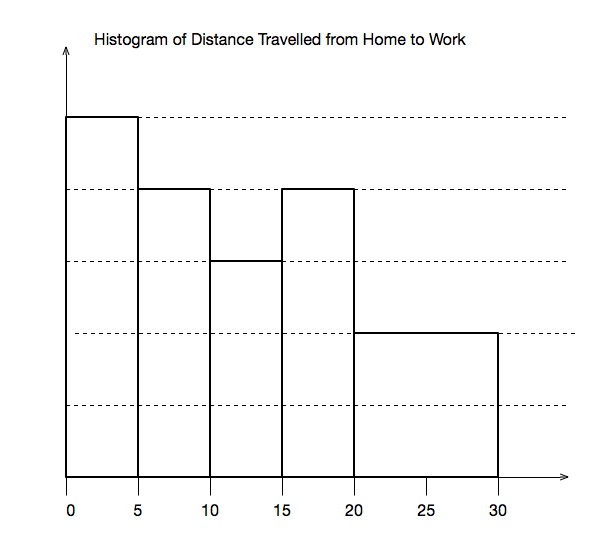The above histogram shows the distance travelled (in miles) from home to work by the inhabitants of a certain town.

If 284 people travelled between 5 and 10 miles, how many people travelled between 20 and 30 miles?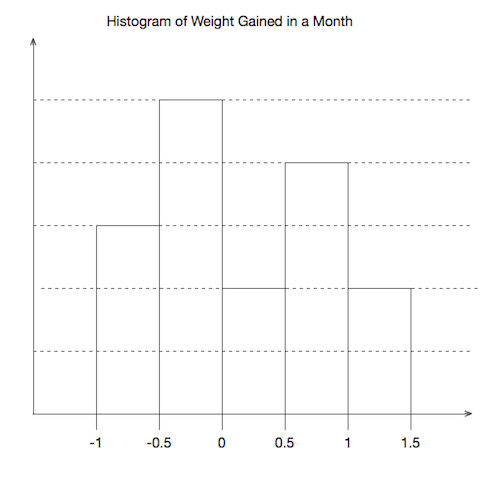The above histogram shows the amount of weight gained by members of a gym in the month of January. If there are 208 members in the gym, how many of them lost weight in January?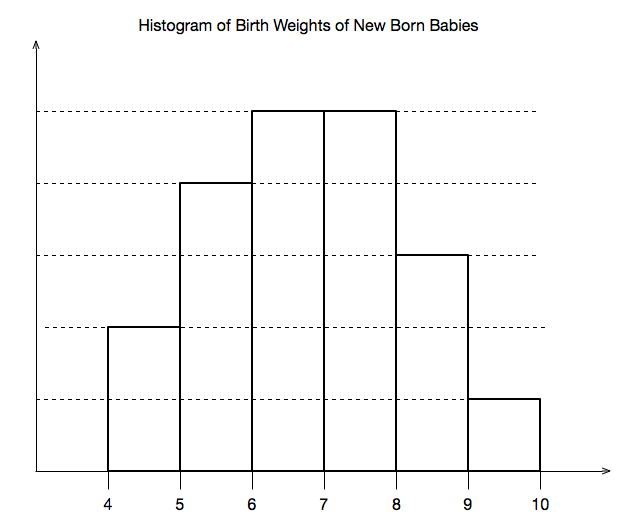The above histogram shows the birth weight (in pounds) of new born babies at a hospital. A baby that is under 5 pounds is considered underweight, and a baby that is over 9 pounds is considered overweight.

What percentage of babies are neither overweight nor underweight?

×